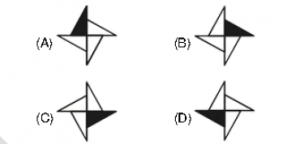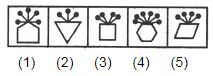# Picture Pattern Classification

#### Video Lesson on Picture Pattern Classification

In the problems of Classification of images, you will be given a set of figures are given where all except one figure will be having similar features. So, You have to find that figure and mark it as an option.

### Example

In The following question four figures have been shown. Out of the four, three figures are similar while one is different in some manner. Find The odd one out.### Solution

In each of the above figures the shaded part is aligned to x-axis or horizontal, except in figure (A) where the shaded part is vertical or aligned to y-axis. Hence figure (A) is odd one out.

### Example

Choose the figure which is different from the rest.### Solution

The pins, equal in number to the number of sides in the main figure are attached to the midpoint of a side of the main figure in case of figures (2), (3), (4) and (5). In fig. (1), these pins are attached to a vertex of the main figure.

### About us

EntryTest.com is a free service for students seeking successful career.CAT - College of Admission Tests. All rights reserved. College of Admission Tests Online Test Preparation The CAT Online